Test: Network Elements - 2

# Test: Network Elements - 2

Test Description

## 15 Questions MCQ Test Network Theory (Electric Circuits) | Test: Network Elements - 2

Test: Network Elements - 2 for Electronics and Communication Engineering (ECE) 2023 is part of Network Theory (Electric Circuits) preparation. The Test: Network Elements - 2 questions and answers have been prepared according to the Electronics and Communication Engineering (ECE) exam syllabus.The Test: Network Elements - 2 MCQs are made for Electronics and Communication Engineering (ECE) 2023 Exam. Find important definitions, questions, notes, meanings, examples, exercises, MCQs and online tests for Test: Network Elements - 2 below.
Solutions of Test: Network Elements - 2 questions in English are available as part of our Network Theory (Electric Circuits) for Electronics and Communication Engineering (ECE) & Test: Network Elements - 2 solutions in Hindi for Network Theory (Electric Circuits) course. Download more important topics, notes, lectures and mock test series for Electronics and Communication Engineering (ECE) Exam by signing up for free. Attempt Test: Network Elements - 2 | 15 questions in 45 minutes | Mock test for Electronics and Communication Engineering (ECE) preparation | Free important questions MCQ to study Network Theory (Electric Circuits) for Electronics and Communication Engineering (ECE) Exam | Download free PDF with solutions
 1 Crore+ students have signed up on EduRev. Have you?
Test: Network Elements - 2 - Question 1

### Which of the following materials has a large number of free electrons in it?

Detailed Solution for Test: Network Elements - 2 - Question 1

The substances which have many free electrons and offer very low resistance are called conductors.

The substances which cannot pass a current under normal conditions are known as insulators. The resistance of an insulator is usually high. Insulators have no free electrons.

Test: Network Elements - 2 - Question 2

### Two bulbs of rating 230 V, 60 W and 230 V, 100 W are connected in parallel across supply mains. Identify the correct statement.

Detailed Solution for Test: Network Elements - 2 - Question 2

Power dissipation in a lamp,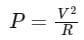Since both bulbs are rated at the same voltage, we can say that resistance of each bulb is inversely proportional to its rated power. Therefore, the resistance of 60 W bulb (R60) is greater than the resistance of 100 W (R100) bulb.
Since lamps connected in parallel, the voltage across the both bulb is same.
The power dissipated across 60 W lamp,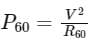The power dissipated across 100 W lamp,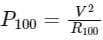As R60 > R100, the power dissipated across 100 W is greater than 60 W.

Hence 100 W bulb will glow brighter in parallel connection.

Test: Network Elements - 2 - Question 3

### The temperature coefficient of resistance of a wire is 0.00125 per °C.  At 300K its resistance is 1 ohm. The resistance of the wire will be 2 ohms at

Detailed Solution for Test: Network Elements - 2 - Question 3

The resistance of most of the metals increases in a linear way with temperature as shown and can be represented by the equation:
RT = R0(1 + αT)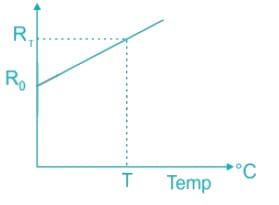RT = Resistance at a temperature T
R0 = Resistance at 0°C
α = Temperature coefficient of the resistance

Calculation:
Given:
α = 0.00125 per °C
R300K = 1 Ω, i.e.
T = 300 - 273 = 27°C
1 = R0(1 + α × 27)   ---(1)
Let the resistance be 2 Ω for some temperature T. We can write:
2 = R0(1 + α T)   ---(2)
(1) ÷ (2)
(1 + α T) = 2 (1 + α × 27)
α (T - 54) = 1
T - 54 = 800
T = 854°C
T = 854 + 273 = 1127 K

Test: Network Elements - 2 - Question 4

Which of the following is the dimension of power?

Detailed Solution for Test: Network Elements - 2 - Question 4

The dimensional formula is defined as the expression of the physical quantity in terms of mass, length, time and ampere.

Explanation-
Power – It is defined as rate of doing work.
∴ P = W/t
Where, P = power, W = work done and t = time.
Now,
Dimensional formula of work (W) = [ML2T-2]

Dimensional formula of time (t) = [T1]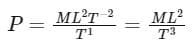∴ The dimensional formula of power P is [ML2T-3].

Test: Network Elements - 2 - Question 5

An ideal current source should have:

Detailed Solution for Test: Network Elements - 2 - Question 5

Ideal voltage source: An ideal voltage source have zero internal resistance.

Practical voltage source: A practical voltage source consists of an ideal voltage source (VS) in series with internal resistance (RS) as follows,

An ideal voltage source and a practical voltage source can be represented as shown in the figure: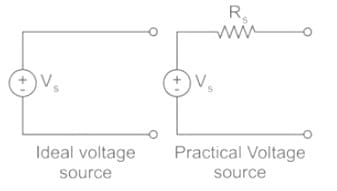Ideal current source: An ideal current source has infinite resistance. Infinite resistance is equivalent to zero conductance. So, an ideal current source has zero conductance.

Practical current source: A practical current source is equivalent to an ideal current source in parallel with high resistance or low conductance.

Ideal and practical current sources are represented as shown in the below figure: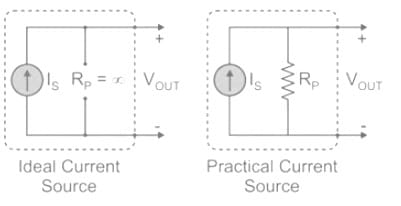Test: Network Elements - 2 - Question 6

Determine the power delivered by the current source in the figure below: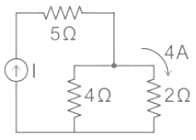Detailed Solution for Test: Network Elements - 2 - Question 6

Power delivered by current source is given by
P = I2 Req
I = current provides by current source
Req = equivalent resistance

Calculation:
Applying the current division rule, we can write: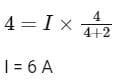Also, the equivalent resistance across the current source is: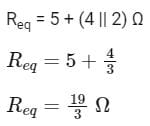Now, the power delivered by the current source will be: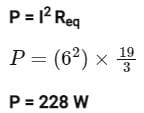Test: Network Elements - 2 - Question 7

In series combination of resistance, the current through each resistance is _________.

Detailed Solution for Test: Network Elements - 2 - Question 7

The measurement of the opposition of the flow of electric current through a conductor is called resistance of that conductor. It is denoted by R.
There are mainly two ways of the combination of resistances:

1. Resistances in series: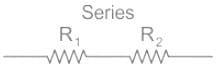• When two or more resistances are connected one after another such that the same current flows through them are called resistances in series.
• The net resistance/equivalent resistance (R) of resistances in series is given by:
• Equivalent resistance, R = R1 + R2
• In series combination of resistance, current is same through each resistance.

2. Resistances in parallel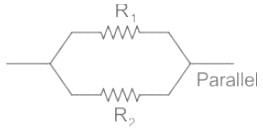• When the terminals of two or more resistances are connected at the same two points and the potential difference across them is equal is called resistances in parallel.
• In parallel combination of resistances, voltage across each resistor is same.
• The net resistance/equivalent resistance(R) of resistances in parallel is given by: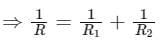Test: Network Elements - 2 - Question 8

A network of resistors is connected to a 16 V battery with an internal resistance of 1 Ω, as shown in the figure. Compute the equivalent resistance of the network.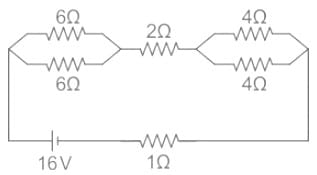Detailed Solution for Test: Network Elements - 2 - Question 8

The circuit after removing the voltage source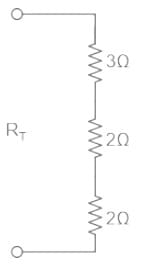Req = Rt = 3 + 2 + 2 = 7 Ω
The equivalent resistance of the network is 7 Ω.

Test: Network Elements - 2 - Question 9

Siemens is the S.I unit of _________.

Detailed Solution for Test: Network Elements - 2 - Question 9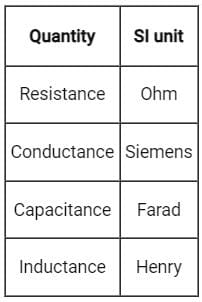Test: Network Elements - 2 - Question 10

If an ideal voltage source and ideal current source are connected in series, the combination

Detailed Solution for Test: Network Elements - 2 - Question 10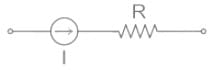In a series circuit, the current flows through all the elements is the same. Thus, any element connected in series with an ideal current source is redundant and it is equivalent to an ideal current source only.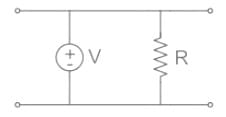In a parallel circuit, the voltage across all the elements is the same. Thus, any element connected in parallel with an ideal voltage source is redundant and it is equivalent to an ideal voltage source only.

Test: Network Elements - 2 - Question 11

The resistance of a coil is 600 Ω. The temperature coefficient at 20°C is 0.1%. What will be the value of resistance at 50°C?

Detailed Solution for Test: Network Elements - 2 - Question 11

Resistance of the material at temperature 'T' °C is given by
RT = RT1[1 + α(T - T1)]
Where,
RT1 = Resistance at temperature 'T1' °C
α = Temperature coefficient of resistance

Calculation:
Given that,
T = 50°C
T1 = 20°C
RT1 = 600 Ω
α = 0.1% = 0.001
Now the value of resistance at 50°C can be calculated as
RT = 600[1 + 0.001(50 - 20)]
RT = 600[1 + 0.001(30)]
RT = 600 + 18
RT = 618 Ω

Test: Network Elements - 2 - Question 12

Which of the following expression correctly represents Kirchhop's Voltage Law (KVL) for the given circuit diagram?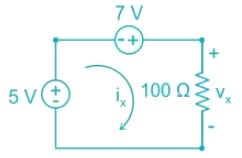Detailed Solution for Test: Network Elements - 2 - Question 12

Concept:

Kirchhoffs Voltage Law (KVL):

• Kirchhoffs Voltage Law or KVL, states that “in any closed loop network, the total voltage around the loop is equal to the sum of all the voltage drops within the same loop” which is also equal to zero.
• In other words, the algebraic sum of all voltages within the loop must be equal to zero i.e. ∑V = 0
• KVL is based on the law of conservation of energy.
• Consider the figure shown below: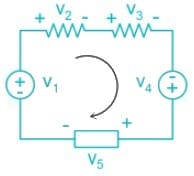∴ According to KVL,
V1 + V2 + V3 + V4 + V5 = 0

Calculation:
The circuit diagram given in the question can be modified asNow by applying KVL in the above circuit, we get
5 + 7 - Vx = 0
∴ - 5 - 7 + Vx = 0

Test: Network Elements - 2 - Question 13

The total effective resistance of the practical capacitor is made of which of the following parameter:

1. Resistance of the lead wires
2. Resistance of the plates
3. Insulation resistance of the dielectric
4. Resistance due to polarisation of the dielectric.
Detailed Solution for Test: Network Elements - 2 - Question 13

Equivalent Circuit for a Capacitor:

• No capacitor is ideal or perfect i.e., electrical energy supplied to a capacitor is not entirely stored in the electric field between the capacitor plates.
• A part of the supplied electrical energy is converted into heat due to the resistances of the various parts of the capacitor.

The total effective resistance is made up of the following resistances:

• Resistance of the lead wires
• Resistance of the plates
• Insulation resistance of the dielectric
• Resistance due to polarisation of the dielectric.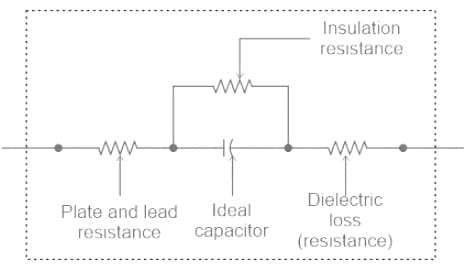Fig. above shows the equivalent circuit of a capacitor. We can replace all the resistances with a single equivalent series resistance Rse as shown in Fig below.

The value of Rse is such that I2R loss in it is equal to the total loss in the capacitor.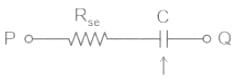Test: Network Elements - 2 - Question 14

Constant voltage source is

Detailed Solution for Test: Network Elements - 2 - Question 14

Active Element:

• The elements that supply energy to the circuit is called active element
• These have an ability to control the flow of charge
• Used for current control and voltage control applications
• Examples: Battery, voltage source, current source, diode

Passive Element:

• The element which receives or absorbs energy and then either converts it into heat (R) or stored it in an electric (C) or magnetic (L) field is called passive element
• Do not need any form of electrical power to operate
• Not able to control the flow of charge
• Used for energy storage, discharge, oscillating, filtering and phase shifting applications
• Examples: Resistor, inductor, capacitor

• Unilateral Element: These elements allow conduction of current in only one direction.
• Example: Diode, transistor, voltage source
• Bilateral Element: These elements allows conduction of current in both directions with same magnitude
• Example: Resistance, inductance, capacitance
Test: Network Elements - 2 - Question 15

Which one of the following is used as an active device in electronic circuits?

Detailed Solution for Test: Network Elements - 2 - Question 15

A network element that is absorbing power i.e. energy delivered to the element is positive then the element is a passive element.

Example: resistor, inductor, and diode.

A network element that is delivering power i.e. energy delivered to the element is negative then the element is an active element.

Example: Op-amp, generator, SCR, etc.

## Network Theory (Electric Circuits)

23 videos|63 docs|60 tests
 Use Code STAYHOME200 and get INR 200 additional OFF Use Coupon Code
Information about Test: Network Elements - 2 Page
In this test you can find the Exam questions for Test: Network Elements - 2 solved & explained in the simplest way possible. Besides giving Questions and answers for Test: Network Elements - 2, EduRev gives you an ample number of Online tests for practice

## Network Theory (Electric Circuits)

23 videos|63 docs|60 tests